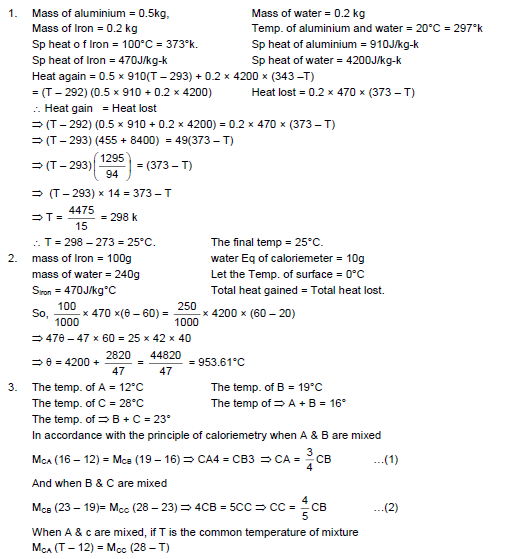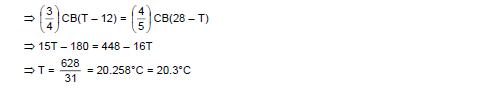# HC Verma Solutions Vol 2 Chapter 3 Calorimetry

HC Verma Solutions Vol 2 Chapter 3 Calorimetry –  Students can download free HC Verma solutions that will help in not only understanding the concepts more clearly but they will be able to improve their problem-solving approach to complex questions given in the chapter. However, before we get into the solutions lets us understand a bit about Calorimetry. It is a word that has been derived from the Latin word Calor and the Greek word Metry where the meaning of calor is heat and metry translates to measure. In general, Calorimetry is defined as the measurement of the amount of heat exchanged.

## Key Calorimetry Topics To Focus On

Some of the topics covered in this chapter include;

• Units of Heat
• Specific Heat Capacity and Molar Heat Capacity
• Specific Latent Heat Of Fusion
• Measurement of Specific Heat and Specific Latent Heat Of Fusion
• Mechanical Equivalent Of Heat

The solutions provided here deal with all the topics mentioned above. Students of 12th grade can extensively make use of this study material to learn the problems given in chapter 3 of the HC Verma book and prepare well for competitive exam like JEE Main.

## Important Questions In Chapter 3

1. Write down the specific heat capacity of melting ice and boiling water.

2. What is the point of keeping a calorimeter in a wooden box where it is insulated thermally from the surroundings? Also, explain why is it necessary to do so?

3. What happens to the temperature when a cold liquid is mixed with a hot liquid in a mixture? Choose the right option.

(a) Temperature first increases then becomes constant (b) Temperature first decreases and then becomes constant (c) Temperature continuously increases (d) Temperature becomes nearly constant.

4. Which quantities will remain constant when two bodies having different temperatures are mixed in a calorimeter?

(a)  the internal energy of each body (b) the total internal energy (c) the sum of the temperatures (d) the total heat

5. What is the ratio of specific heat capacity to the molar heat capacity of a body related to?

(a) is dimensionless (b) is a universal constant (c) depends on the molecular weight of the body (d) depends on the mass of the body

## HC Verma Solutions Vol 2 Calorimetry Chapter 3